# Mensuration

Mensuration is a branch of mathematics concerned with the calculation of geometric figures and their parameters such as weight, volume, form, surface area, lateral surface area, and so on. At GeeksforGeeks, you can learn about mensuration in a very easy manner. Principles of mensuration along with all the essential formulas of mensuration are discussed here.

For a better understanding of these ideas, the properties of various geometric forms and the accompanying figures are also shown. Mensuration is a branch of mathematics that deals with the scale, volume, or area of various geometric forms. These forms are available in two or three dimensions.

Table of Content

## Difference Between 2D and 3D shapes

2D Shape 3D Shape
Any shape is 2D if it is bound by three or more straight lines in a plane. A shape is a three-dimensional shape if there are several surfaces or planes around it.
There is no height or depth in these shapes. In contrast to 2D forms, these are sometimes known as solid shapes and have height or depth.
These shapes just have length and width as their dimensions. Since they have depth (or height), breadth, and length, they are referred to as three-dimensional objects.
We can calculate their perimeter and area. Their volume, curved surface area, lateral surface area, or total surface area can all be calculated.

## Mensuration in Maths-Terminology

Terms Abbreviation Unit Definition
Area A m2 or cm2 The surface that the closed form covers is known as the area.
Perimeter P cm or m A perimeter is the length of the continuous line that encircles the specified figure.
Volume V cm3 or m3 A 3D shape’s space is referred to as its volume.
Curved Surface Area CSA m2 or cm2 The overall area is known as a Curved surface area if there is a curved surface. Example: Sphere
Lateral Surface area LSA m2 or cm2 The term “Lateral Surface area” refers to the combined area of all lateral surfaces that encircle the provided figure.
Total Surface Area TSA m2 or cm2 The total surface area is the total of all the curved and lateral surface areas.
Square Unit m2 or cm2 A square unit is the area that a square of side one unit covers.
Cube Unit m3 or cm3 The space taken up by a cube with a single side.

## Mensuration of 2D shapes

Mensuration is the measuring theory. It is the field of mathematics that is used to measure various figures such as cube, cuboid, square, rectangle, cylinder, and so on. Mensuration of two-dimensional figures such as area, perimeter, and so on. The form or figure having two dimensions, such as length and breadth, is referred to as a 2-D form. For example, square, rectangle, triangle, parallelogram, trapezium, rhombus, and so on. We can measure 2-D forms using Area (A) and Perimeter (P) as discussed below:

## Mensuration of 3D shapes

Mensuration is concerned with the measuring of three-dimensional solids in terms of total surface area, lateral/curved surface area, and volume. 3D figures are those that have more than two dimensions, such as length, breadth, and height. Cube, Cuboid, Sphere, Cylinder, Cone, and other three-dimensional forms are examples. Total Surface Area, Lateral Surface Area, Curved Surface Area, and Volume are used to calculate the 3D figure are discussed in the articles below:

## Mensuration Formulas For 2D Shapes

 Shape Area (Square units) Perimeter (units) Figure Square a2 4a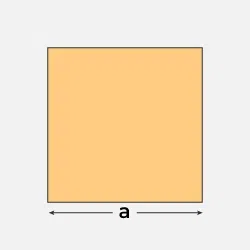Rectangle l × b 2 (l + b)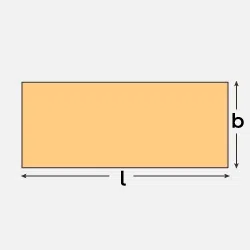Circle πr2 2 π r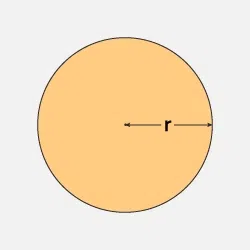Scalene Triangle √[s(s−a)(s−b)(s−c)],Where, s = (a+b+c)/2 a+b+c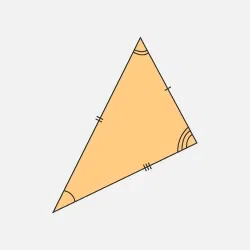Isosceles Triangle ½ × b × h 2a + b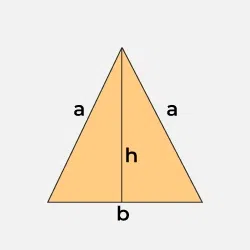Equilateral triangle (√3/4) × a2 3a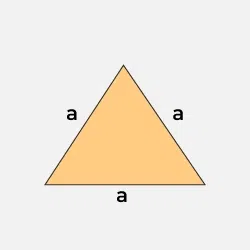Right Angle Triangle ½ × b × h b + hypotenuse + h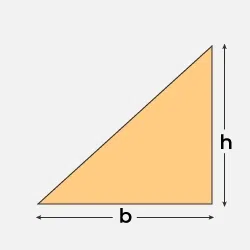Rhombus ½ × d1 × d2 4 × side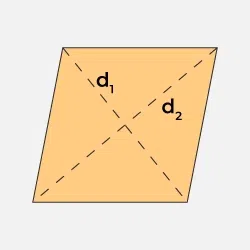Parallelogram b × h 2(l+b)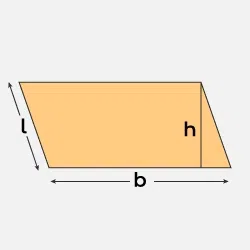Trapezium ½ h(a+c) a+b+c+d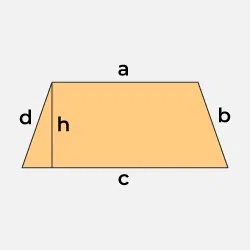## Mensuration Formulas for 3D Shapes

 Shape Volume (Cubic units) Curved Surface Area (CSA) or Lateral Surface Area (LSA) (Square units) Total Surface Area (TSA) (Square units) Figure Cube a3 LSA = 4 a2 6 a2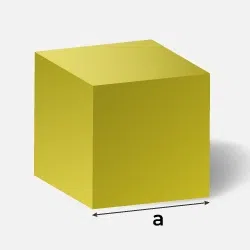Cuboid l × b × h LSA = 2h(l + b) 2 (lb +bh +hl)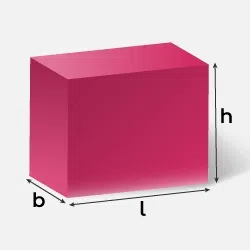Sphere (4/3) π r3 4 π r2 4 π r2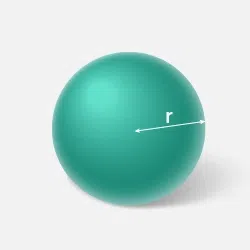Hemisphere (⅔) π r3 2 π r2 3 π r2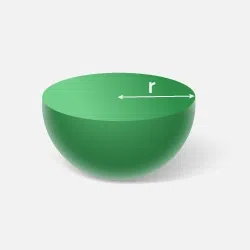Cylinder π r 2 h 2π r h 2πrh + 2πr2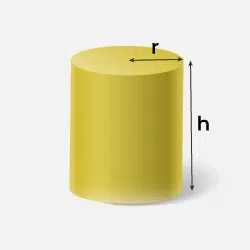Cone (⅓) π r2 h π r l πr (r + l)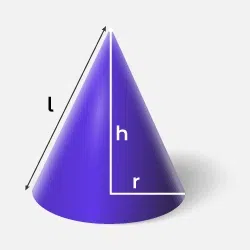## Solved Problems on Mensuration

Problem 1: Find the volume of a cone if the radius of its base is 1.5 cm and its perpendicular height is 5 cm.

Solution:

Radius of the cone, r = 1.5 cm

Height of the cone, h = 5 cm

∴ Volume of the cone, V = 13πr2h=13×227×(1.5)2×5= 11.79 cm3

Thus, the volume of the cone is 11.79 cm3

Problem 2: The dimensions of a cuboid are 44 cm, 21 cm, 12 cm. It is melted and a cone of height 24 cm is made. Find the radius of its base.

Solution:

The dimensions of the cuboid are 44 cm, 21 cm and 12 cm.

Let the radius of the cone be r cm.

Height of the cone, h = 24 cm

It is given that cuboid is melted to form a cone.

∴ Volume of metal in cone = Volume of metal in cuboid

⇒(1/3)πr2h=44×21×12

⇒(1/3)×(22/7)×r2×24=44×21×12

⇒r= √(44×21×12×21) / (22×24)

=21 cm

Thus, the radius of the base of cone is 21 cm.

Problem 3: The radii of two circular ends of frustum shape bucket are 14 cm and 7 cm. The height of the bucket is 30 cm. How many liters of water can it hold? (1 litre = 1000 cm3)

Solution:

Radius of one circular end, r1 = 14 cm

Radius of other circular end, r2 = 7 cm

Height of the bucket, h = 30 cm

∴ Volume of water in the bucket = Volume of frustum of cone
=(1/3)πh(r12+r1r2+r22)

=13×22/7×30×(142+14×7+72)

=13×22/7×30×343=10780 cm3

=107801000=10.780 L

Thus, the bucket can hold 10.780 litres of water.

Problem 4: How many bricks each measuring 64 cm x 11.25 cm x 6 cm. will be needed to build a wall measuring 8m x 3m x 22.5m?

Solution:

Volume of wall = V1
Volume of Brick= V2
Number of Bricks =V1/V2
=(8m*3m*22.5)/(64cm*11.25cm*6cm)
=(800*300*22.5)/(64*11.25*6)
= 125000

## FAQs on Mensuration

### Q1: What is Mensuration?

Mensuration is a branch of mathematics concerned with calculation of geometric figures and their parameters such as weight, volume, form, surface area, lateral surface area, and so on.

### Q2: What are 2D and 3D shapes?

Any shape is considered to be 2D if it is bound by three or more straight lines in a plane whereas a shape is a three-dimensional shape if there are several surfaces or planes around it.